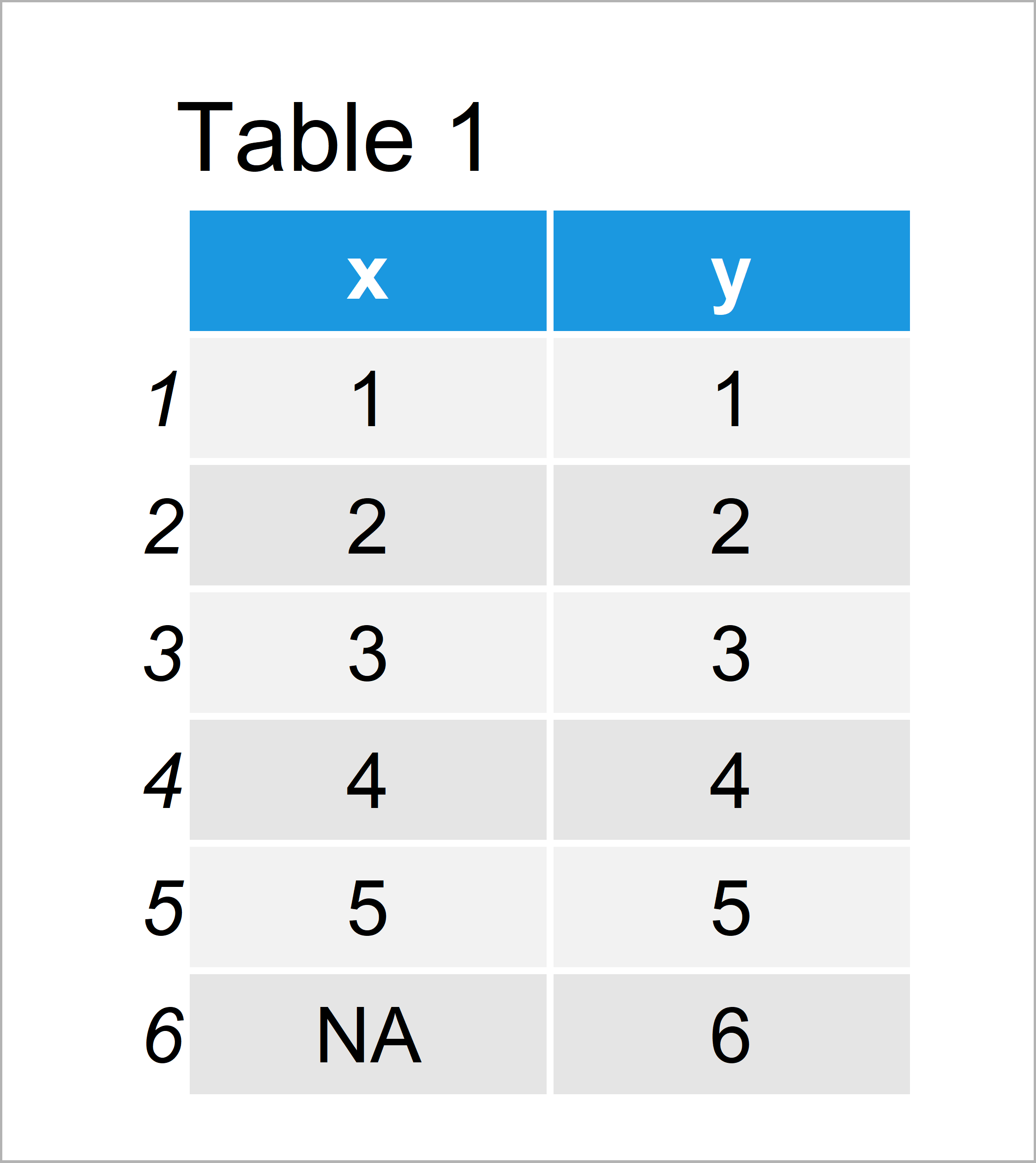# Specify Multiple Arguments in apply Functions in R (Example)

In this tutorial you’ll learn how to pass several parameters to the family of apply functions in the R programming language.

The page will consist of this information:

Here’s the step-by-step process!

## Creation of Example Data

We use the following data as basement for this R tutorial:

```data <- data.frame(x = c(1:5, NA),    # Example data
y = c(1:6))
data                                  # Print example data```Have a look at the table that has been returned after running the previous R code. It shows that our example data has six rows and two variables. Note that the last data cell in the first variable contains an NA value.

## Example: Passing Several Arguments to FUN of apply() Functions Using …

In this example, I’ll show how to use multiple parameters within the apply function.

First, let’s use the apply function without any additional parameters:

```apply(data, 2, mean)                  # apply() without additional arguments
#   x   y
#  NA 3.5```

As you can see based on the previous output of the RStudio console, the output for the column x is NA. The reason for this is the NA value in the sixth row of our data frame.

Fortunately, the mean function (i.e. the function that we have used within the apply function) provides the na.rm argument.

So how can we use this additional argument within apply? The answer is given in the help documentation of the apply function:

apply(X, MARGIN, FUN, …)
… optional arguments to FUN.

In other words: we can simply add as many additional arguments within the apply function by simply specifying them separated by a comma.

Let’s do this in practice:

```apply(data, 2, mean, na.rm = TRUE)    # apply() with additional argument
#   x   y
# 3.0 3.5```

The RStudio console shows our updated result. As you can see, the output for the x variable is not NA anymore.

In this example I have illustrated how to pass additional arguments within the apply function. However, please note that we could use the same kind of logic for other functions of the apply family (e.g. lapply, sapply, and mapply).

## Video, Further Resources & Summary

In case you need further info on the R code of this post, I recommend watching the following video of my YouTube channel. In the video, I show the topics of this article in a live session.

In addition, you may want to have a look at the related tutorials on Statistics Globe.

To summarize: This tutorial has illustrated how to use multiple arguments within apply() in the R programming language. If you have any further questions or comments, tell me about it in the comments below. Furthermore, don’t forget to subscribe to my email newsletter to receive regular updates on new tutorials.

Subscribe to the Statistics Globe Newsletter